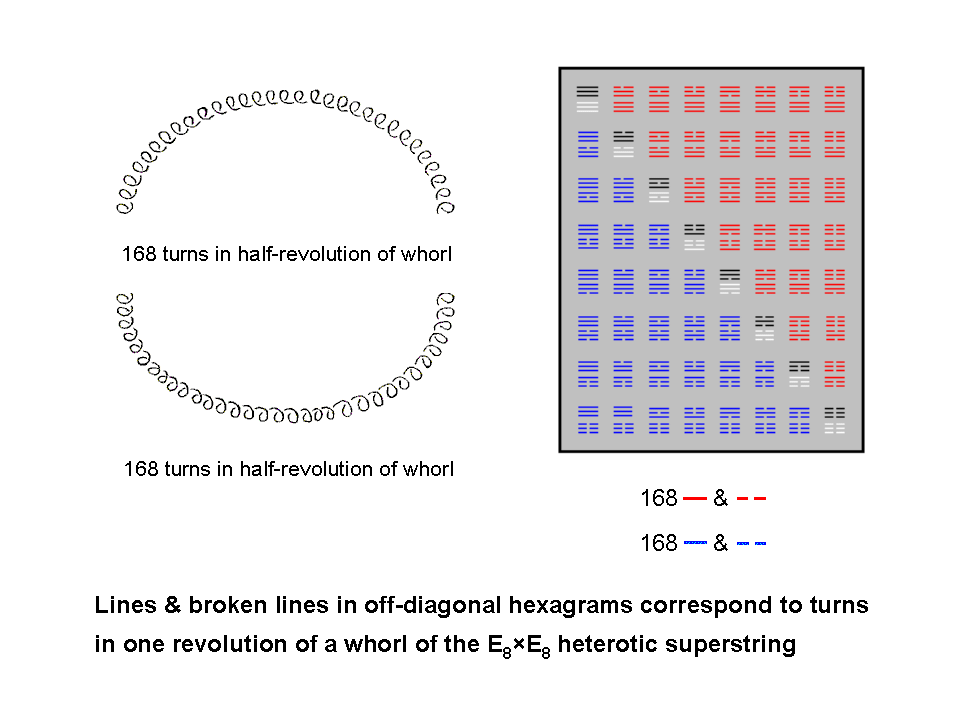Home

 << Previous      2  3  4    Next >>

The I Ching table of 64 hexagramsThe 28 red hexagrams above the eight diagonal hexagrams in the 8×8 array of 64 hexagrams comprise 168 lines & broken lines, 84 in each set of 56 trigrams. The latter are seven copies of the basic set of eight trigrams containing 12 lines & 12 broken lines. Similarly for the 28 blue hexagrams below the diagonal. The 336 off-diagonal lines & broken lines therefore exhibit the following divisions:

336 = 168 + 168.
168 = 84 + 84 = 7×24.
24 = 12 + 12.

Compare this with the 336 helical turns made in a whorl as it winds once around the spin-axis of the UPA/superstring. A half-revolution in its outer half comprises 168 turns and a half-revolution in its inner half comprises another 168 turns. There are 84 turns in each quarter-revolution. The off-diagonal lines & broken lines correspond to helical turns — circularly polarized oscillations of a standing wave. The two off-diagonal halves of the table of hexagrams express the inner and outer half-revolutions of a whorl, each containing 168 oscillations. Notice that, although the 56 off-diagonal hexagrams contain 168 (Yang) lines and 168 (Yin) broken lines, this division does not correspond to the distinction between the outer and inner halves of the superstring. This is because this geometrical feature corresponds to the mirror-reflection across the diagonal of the 8×8 array, and the 56 trigrams in each off-diagonal half contain 84 lines and 84 broken lines, as they are seven copies of the eight basic trigrams, which contain 12 lines and 12 broken lines.

 << Previous      2  3  4    Next >>

Home